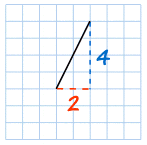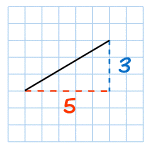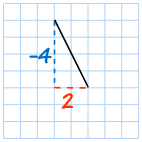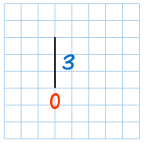# Slope (Gradient) of a Straight Line

The Slope (also called Gradient) of a straight line shows how steep a straight line is.

## Calculate

To calculate the Slope:

Divide the change in height by the change in horizontal distance
 Slope = Change in YChange in XHave a play (drag the points):

## Examples:The Slope of this line = 3 3 = 1 So the Slope is equal to 1The Slope of this line = 4 2 = 2 The line is steeper, and so the Slope is larger.The Slope of this line = 3 5 = 0.6 The line is less steep, and so the Slope is smaller.

## Positive or Negative?

Going from left-to-right, the cyclist has to Push on a Positive Slope:When measuring the line:

• Starting from the left and going across to the right is positive
(but going across to the left is negative).
• Up is positive, and down is negativeSlope = −4 2 = −2

That line goes down as you move along, so it has a negative Slope.

## Straight AcrossSlope = 0 5 = 0

A line that goes straight across (Horizontal) has a Slope of zero.

## Straight Up and DownSlope = 3 0 = undefined

That last one is a bit tricky ... you can't divide by zero,
so a "straight up and down" (vertical) line's Slope is "undefined".

## Rise and Run

Sometimes the horizontal change is called "run", and the vertical change is called "rise" or "fall":They are just different words, none of the calculations change.